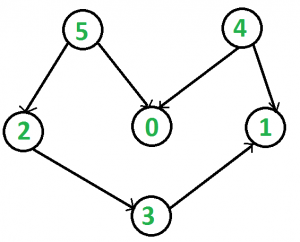# Lexicographically Smallest Topological Ordering

Given a directed graph with N vertices and M edges that may contain cycles, the task is to find the lexicographically smallest topological ordering of the graph if it exists otherwise print -1 (if the graph has cycles).
Lexigraphically smallest topological ordering means that if two vertices in a graph do not have any incoming edge then the vertex with the smaller number should appear first in the ordering.
For Example, in the image below many topological orderings are possible e.g 5 2 3 4 0 1, 5 0 2 4 3 1.
But the smallest ordering is 4 5 0 2 3 1.

Examples:

Input:Output: 4 5 0 2 3 1
Even though 5 4 0 2 3 1 is also a valid topological
ordering of the given graph but it is not
lexicographically smallest.

## Recommended: Please try your approach on {IDE} first, before moving on to the solution.

Approach: We will use Kahn’s algorithm for Topological Sorting with a modification. Instead of using a queue we will use a multiset to store the vertices to make sure that every time we pick a vertex it is the smallest possible of all. The overall Time complexity changes toBelow is the implementation of the above approach:

 `// C++ implementation of the approach ` `#include ` `using` `namespace` `std; ` ` `  `// Class to represent a graph ` `class` `Graph { ` `    ``int` `V; ``// No. of vertices' ` ` `  `    ``// Pointer to an array containing ` `    ``// adjacency listsList ` `    ``list<``int``>* adj; ` ` `  `public``: ` `    ``Graph(``int` `V); ``// Constructor ` ` `  `    ``// Function to add an edge to graph ` `    ``void` `addEdge(``int` `u, ``int` `v); ` ` `  `    ``// Function to print the required topological ` `    ``// sort of the given graph ` `    ``void` `topologicalSort(); ` `}; ` ` `  `// Constructor ` `Graph::Graph(``int` `V) ` `{ ` `    ``this``->V = V; ` `    ``adj = ``new` `list<``int``>[V]; ` `} ` ` `  `// Function to add an edge to the graph ` `void` `Graph::addEdge(``int` `u, ``int` `v) ` `{ ` `    ``adj[u].push_back(v); ` `} ` ` `  `// Function to print the required topological ` `// sort of the given graph ` `void` `Graph::topologicalSort() ` `{ ` `    ``// Create a vector to store indegrees of all ` `    ``// the vertices ` `    ``// Initialize all indegrees to 0 ` `    ``vector<``int``> in_degree(V, 0); ` ` `  `    ``// Traverse adjacency lists to fill indegrees of ` `    ``// vertices ` `    ``// This step takes O(V+E) time ` `    ``for` `(``int` `u = 0; u < V; u++) { ` `        ``list<``int``>::iterator itr; ` `        ``for` `(itr = adj[u].begin(); itr != adj[u].end(); itr++) ` `            ``in_degree[*itr]++; ` `    ``} ` ` `  `    ``// Create a set and inserting all vertices with ` `    ``// indegree 0 ` `    ``multiset<``int``> s; ` `    ``for` `(``int` `i = 0; i < V; i++) ` `        ``if` `(in_degree[i] == 0) ` `            ``s.insert(i); ` ` `  `    ``// Initialize count of visited vertices ` `    ``int` `cnt = 0; ` ` `  `    ``// Create a vector to store result (A topological ` `    ``// ordering of the vertices) ` `    ``vector<``int``> top_order; ` ` `  `    ``// One by one erase vertices from setand insert ` `    ``// adjacents if indegree of adjacent becomes 0 ` `    ``while` `(!s.empty()) { ` ` `  `        ``// Extract vertex with minimum number from multiset ` `        ``// and add it to topological order ` `        ``int` `u = *s.begin(); ` `        ``s.erase(s.begin()); ` `        ``top_order.push_back(u); ` ` `  `        ``// Iterate through all its neighbouring nodes ` `        ``// of erased node u and decrease their in-degree ` `        ``// by 1 ` `        ``list<``int``>::iterator itr; ` `        ``for` `(itr = adj[u].begin(); itr != adj[u].end(); itr++) ` ` `  `            ``// If in-degree becomes zero, add it to queue ` `            ``if` `(--in_degree[*itr] == 0) ` `                ``s.insert(*itr); ` ` `  `        ``cnt++; ` `    ``} ` ` `  `    ``// Check if there was a cycle ` `    ``if` `(cnt != V) { ` `        ``cout << -1; ` `        ``return``; ` `    ``} ` ` `  `    ``// Print topological order ` `    ``for` `(``int` `i = 0; i < top_order.size(); i++) ` `        ``cout << top_order[i] << ``" "``; ` `} ` ` `  `// Driver code ` `int` `main() ` `{ ` `    ``// Create the graph ` `    ``Graph g(6); ` `    ``g.addEdge(5, 2); ` `    ``g.addEdge(5, 0); ` `    ``g.addEdge(4, 0); ` `    ``g.addEdge(4, 1); ` `    ``g.addEdge(2, 3); ` `    ``g.addEdge(3, 1); ` ` `  `    ``// Find the required topological order ` `    ``g.topologicalSort(); ` ` `  `    ``return` `0; ` `} `

Output:

```4 5 0 2 3 1
```My Personal Notes arrow_drop_upCheck out this Author's contributed articles.

If you like GeeksforGeeks and would like to contribute, you can also write an article using contribute.geeksforgeeks.org or mail your article to contribute@geeksforgeeks.org. See your article appearing on the GeeksforGeeks main page and help other Geeks.

Please Improve this article if you find anything incorrect by clicking on the "Improve Article" button below.